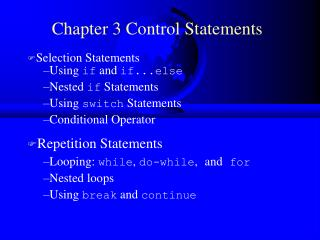Download PresentationChapter 3 Control Statements

# Chapter 3 Control Statements

Download Presentation## Chapter 3 Control Statements

- - - - - - - - - - - - - - - - - - - - - - - - - - - E N D - - - - - - - - - - - - - - - - - - - - - - - - - - -
##### Presentation Transcript

1. Selection Statements • Using if and if...else • Nested if Statements • Using switch Statements • Conditional Operator • Repetition Statements • Looping: while, do-while,and for • Nested loops • Using break and continue Chapter 3 Control Statements

2. Repetitions • while Loops • do-while Loops • for Loops • break and continue

3. while Loop Flow Chart while (continuation-condition) { // loop-body; }

4. while Loop Flow Chart, cont. int i = 0; while (i < 100) { System.out.println( "Welcome to Java!"); i++; }

5. Example 3.2: Using while Loops // TestWhile.java: Test the while loop import javax.swing.JOptionPane; public class TestWhile { /** Main method */ public static void main(String[] args) { int data; int sum = 0; // Read an initial data String dataString = JOptionPane.showInputDialog(null, "Enter an int value, \nthe program exits if the input is 0", "Example 3.2 Input", JOptionPane.QUESTION_MESSAGE); data = Integer.parseInt(dataString); // Keep reading data until the input is 0 while (data != 0) { sum += data; // Read the next data dataString = JOptionPane.showInputDialog(null, "Enter an int value, \nthe program exits if the input is 0", "Example 3.2 Input", JOptionPane.QUESTION_MESSAGE); data = Integer.parseInt(dataString); } JOptionPane.showMessageDialog(null, "The sum is " + sum, "Example 3.2 Output", JOptionPane.INFORMATION_MESSAGE); System.exit(0); } }

6. Caution Don’t use floating-point values for equality checking in a loop control. Since floating-point values are approximations, using them could result in imprecise counter values and inaccurate results. This example uses int value for data. If a floating-point type value is used for data, (data != 0) may be true even though data is 0. // data should be zero double data = Math.pow(Math.sqrt(2), 2) - 2; if (data == 0) System.out.println("data is zero"); else System.out.println("data is not zero");

7. do-while Loop do { // Loop body; } while (continue-condition);

8. for Loops for (initial-action; loop-continuation-condition; action-after-each-iteration) { //loop body; } int i = 0; while (i < 100) { System.out.println("Welcome to Java! ” + i); i++; } Example: int i; for (i = 0; i < 100; i++) { System.out.println("Welcome to Java! ” + i); }

9. for Loop Flow Chart for (initial-action; loop-continuation-condition; action-after-each-iteration) { //loop body; }

10. for Loop Example int i; for (i = 0; i<100; i++) { System.out.println( "Welcome to Java"); }

11. Which Loop to Use? The three forms of loop statements, while, do, and for, are expressively equivalent; that is, you can write a loop in any of these three forms. I recommend that you use the one that is most intuitive and comfortable for you. In general, a for loop may be used if the number of repetitions is known, as, for example, when you need to print a message 100 times. A while loop may be used if the number of repetitions is not known, as in the case of reading the numbers until the input is 0. A do-while loop can be used to replace a while loop if the loop body has to be executed before testing the continuation condition.

12. Caution Adding a semicolon at the end of the for clause before the loop body is a common mistake, as shown below: for (int i=0; i<10; i++); { System.out.println("i is " + i); } Wrong

13. Caution, cont. Wrong Similarly, the following loop is also wrong: int i=0; while (i<10); { System.out.println("i is " + i); i++; } In the case of the do loop, the following semicolon is needed to end the loop. int i=0; do { System.out.println("i is " + i); i++; } while (i<10); Correct

14. The break Keyword

15. The continue Keyword

16. Using break Example for using the break keyword: 3.5 // TestBreak.java: Test the break keyword in the loop public class TestBreak { /** Main method */ public static void main(String[] args) { int sum = 0; int item = 0; while (item < 5) { item ++; sum += item; //if (sum >= 6) break; } System.out.println("The sum is " + sum); } }

17. Using continue Example for using the continue keyword: // TestContinue.java: Test the continue keyword public class TestContinue { /** Main method */ public static void main(String[] args) { int sum = 0; int item = 0; while (item < 5) { item++; if (item == 2) continue; sum += item; } System.out.println("The sum is " + sum); } }

18. Review of Terms: Deena’s Class • The 3 essential elements of a programming loop: priming, test, and update • User-controlled loops vs Program-controlled loops • Some user-controlled loops use sentinels. • Accumulator and Marker variables: how are these used in loops? • What is a flag variable? When do you use it?